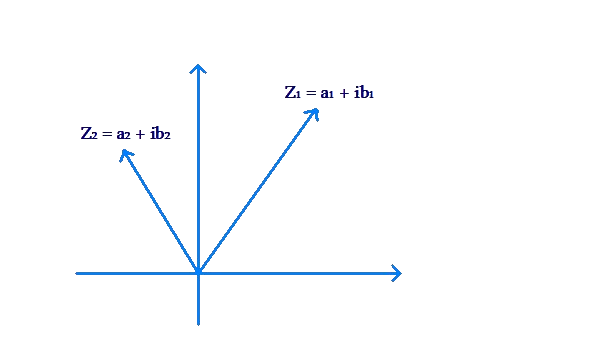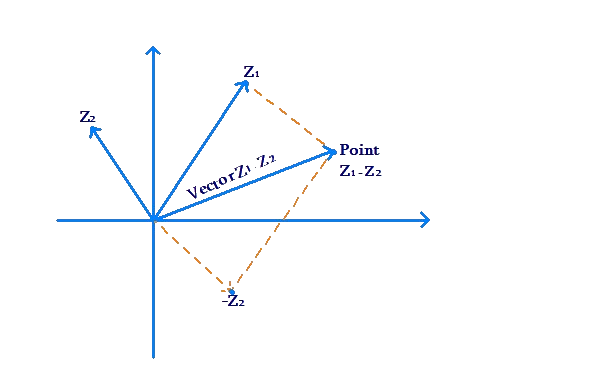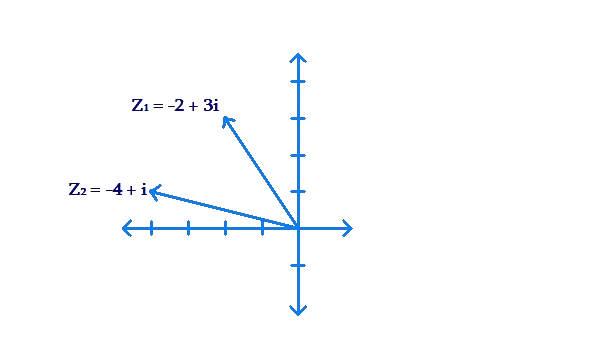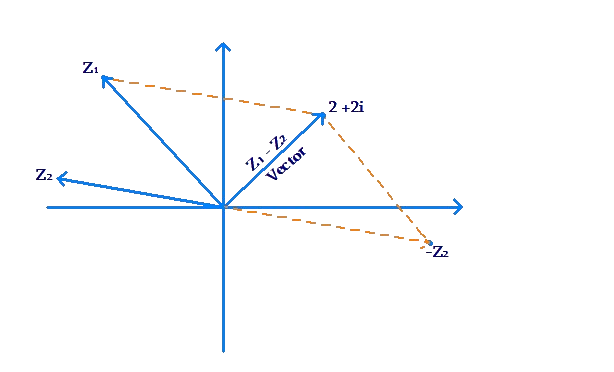# Subtraction

Go back to  'Complex-Numbers'

As you may expect now, the subtraction of complex numbers is geometrically similar to vector subtraction. Once again, we take two complex numbers $${z_1}$$ and $${z_2}$$:

\begin{align}&{z_1} = {a_1} + i{b_1}\\&{z_2} = {a_2} + i{b_2}\end{align}

To geometrically evaluate $${z_1} - {z_2}$$, we first plot these two numbers in the complex plane, and treat them as vectors:We need to find $${z_1} - {z_2}$$, or $${z_1} + \left( { - {z_2}} \right)$$. In other words, we need to find the vector sum of $${z_1}$$ and $$- {z_2}$$. Thus, we reverse $${z_2}$$ (to obtain $$- {z_2}$$), and then add $${z_1}$$ and $$- {z_2}$$ using the parallelogram law of vector addition:The resultant vector represents the vector $${z_1} - {z_2}$$, while its tip represents the point $${z_1} - {z_2}$$.

Example 1: From $${z_1} = - 2 + 3i$$, subtract $${z_2} = - 4 + i$$. Solution. First, we plot these two points in the plane, and treat them as vectors:Then, we reverse vector $${z_2}$$, and add $${z_1}$$ and $$- {z_2}$$ using the parallelogram law:The resultant vector represents $${z_1} - {z_2}$$, while its tip represents the point $${z_1} - {z_2}$$, which is $$2 + 2i$$.

We can verify this subtraction result algebraically:

\begin{align}&{z_1} - {z_2} = \left( { - 2 + 3i} \right) - \left( { - 4 + i} \right)\\&= \left( { - 2 + 4} \right) + i\left( {3 - 1} \right) = 2 + 2i\end{align}

Complex Numbers
grade 10 | Questions Set 1
Complex Numbers
Complex Numbers
Complex Numbers
grade 10 | Questions Set 2